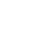0

# Newton’s Fractal (which Newton knew nothing about)Newton’s Fractal (which Newton knew nothing about)

The Newton fractal is a boundary set in the complex plane which is characterized by Newton’s method applied to a fixed polynomialor transcendental function. It is the Julia set of the meromorphic functionwhich is given by Newton’s method. When there are no attractive cycles (of order greater than 1), it divides the complex plane into regions, each of which is associated with a rootof the polynomial,. In this way the Newton fractal is similar to the Mandelbrot set, and like other fractals it exhibits an intricate appearance arising from a simple description. It is relevant to numerical analysis because it shows that (outside the region of quadratic convergence) the Newton method can be very sensitive to its choice of start point.

From Newton’s method to Newton’s fractal (which Newton knew nothing about)

## Like it? Share with your friends!

0hate
0
hateconfused
0
confusedfail
0
failfun
0
fungeeky
0
geekylove
0
lovelol
0
lolomg
0
omgwin
0
win